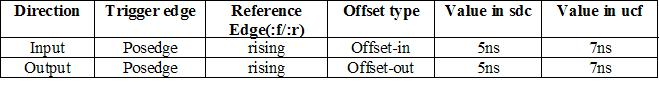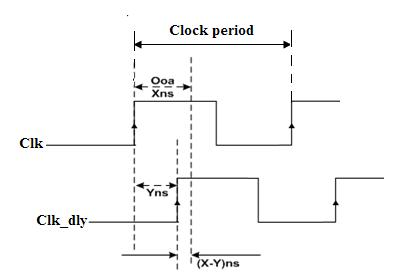# AR# 51048

|

## 説明

I specified define_input_delay in Synplifys SDC file.

How does Synplify forward annotate OFFSET constraints in UCF?

## ソリューション

Please refer to SolvNet Doc 026883 for more information on this topic.

Synplify Pro supports specification of I/O offset constraints in the form of input/output delays.

These constraints are forward annotated as offset in/out in the .ucf file for the place and route tool.

However, there is a difference between the value specified in the .sdc file and the value forward annotated to the .ucf file.

The offset value forward annotated to the .ucf file is different from the I/O-delay value specified in the .sdc file.

Offset calculations for Xilinx:

Offset-in can be specified in two ways, offset-in before and offset-in after.

Similarly offset-out can be defined as offset-out after and offset-out before.

'Before' means, before the capture edge of the destination.

'After' means, after the launch edge of the source.

Defining Offset IN/OUT Before/After:

Oia - Offset-in after which is the delay from input device to the FPGA input pin
Oib - Offset-in before which is the delay from FPGA input pin/pad to the primary input f/f.
Ooa - Offset-out after which is the delay from primary output f/f to the output pin/pad.
Oob - Offset-out before which is the delay from FPGA output pin/pad to the output device.

The data coming from the input device to the FPGA has an available time of one clock period.

To satisfy the timing for the input/output:
Oib + Oia = clock period  -(1)
Oob + Ooa = clock period  -(2)

The offset values entered into the .sdc file are offset-in after (Oia) and offset-out before (Oob).

The values forwarded to the .ucf file are offset-in before (Oib) and offset-out after (Ooa).

Accordingly, the Oib and Ooa values are calculated from Oia and Oob as follows:
Oib = clock period - Oia  -(3)
Ooa = clock period - Oob  -(4)

This is the reason for the difference in values entered into the .sdc file and the values forward annotated to the .ucf file.

Both are equivalent, but expressed in different ways.

Note that the above is true when the input/output is rising-edge triggered.

Practical observation:

Clock period = 12nsSDC constraint:

define_input_delay  -default  5.00 -route 0.00 -ref {clk:r}
define_output_delay -default  5.00 -route 0.00 -ref {clk:r}

UCF constraint:

OFFSET = OUT : 7.000 : AFTER clk ;
OFFSET = IN  : 7.000 : BEFORE clk ;

What is the role of phase-shift in offset calculations?

As phase is considered for the clock triggering of the input/output flip-flop, the role of the clock phase with respect to Oib and Ooa is explained.

For Offset-in:
Consider an offset-in before (Oib) of X ns specified with respect to clock 'Clk'.

Now if 'Clk' is delayed or phase shifted right by Y ns (Clk_dly), the triggering edge at the receiver is moving Y ns away from the triggering edge of the sender so that the new Oib becomes Oib + Y ns.Accordingly, equation (3) can be written as,

Oib + Yns = clock period - Oia so
Oib       = clock period - Oia - Y  --(5)

For Offset-out:

Consider an offset-out after (Ooa) of X ns specified with respect to clock 'Clk'.

Now if 'Clk' is delayed or phase shifted right by Y ns (Clk_dly), the triggering edge at the sender is moving Y ns closer to the triggering edge at the receiver so that new Ooa becomes Ooa - Y ns.Accordingly, equation (4) can be written as,

Ooa - Yns = clock period - Oob, or
Ooa       = clock period - Oob + Y  --(6)

From equations (5) and (6) we see that any phase shift in the clock is added to offset-out and subtracted from offset-in.

To validate equations (5) and (6), consider the offset on a negative-edge triggered flop.

Unlike positive-edge triggered inputs/outputs, the calculation of offset for forward annotation is different for negative-edge triggered input/outputs.

When the input or output is falling-edge triggered, the offset values are calculated as follows:

Oib = clock period  - Oia  - (clock period/2)  -(7)
Ooa = clock period  - Oob  + (clock period/2)  -(8)

That is, half the period is subtracted for the offset-in calculation and added for offset-out calculation.

The reason for the half period subtraction or addition is because any phase difference induced at the input or output flip-flop is also considered for offset calculation.

Now, a negative-edge triggered flip-flop is nothing but a half period phase shifted clock:

Y = clock period/2     -- (9)

For input:

Substituting equation (9) in (5) gives

Oib = clock period - Oia - (clock period/2)

which is essentially equation (7) - the offset-in forward annotated for negative-edge triggered inputs.

For output:

Substituting equation (9) in equation (6) gives

Ooa = clock period - Oob + (clock period/2)

which is essentially equation (8) - the offset-out forward annotated for negative-edge triggered outputs.

Accordingly, half a clock period is subtracted or added to offset-in and offset-out when forward annotated to the ucf file.

Practical observations:
Clock period = 12nsSDC constraint:

define_input_delay  -default  5.00 -route 0.00 -ref {clk:f}
define_output_delay -default  5.00 -route 0.00 -ref {clk:f}

UCF constraint:

OFFSET = IN  : 1.000  : BEFORE clk ;
OFFSET = OUT : 13.000 : AFTER clk ;

AR# 51048

ステータス アクティブ

デバイス
ツール
People Also Viewed